Next: How to use a Up: Output Compare Interrupts Previous: Declaring the ISR's Interrupt

## What is Pulse Width Modulation?

Pulse width modulation or PWM is a standard way by which a digital device can generate an analog voltage. This section discusses how you can use the MicroStamp11 to generate a PWM signal that can be interfaced to a simple capacitive circuit and thereby generate an analog voltage.

Let's define a signal as a function that maps time onto some real number. Consider, for instance, a two-terminal electronic device. The voltage over this device at time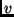is denoted as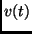. We can think ofas mapping the timeto the voltage.

We say a signal,is periodic if there exists a positive time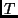such that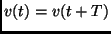for all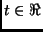. In other words, at any moment,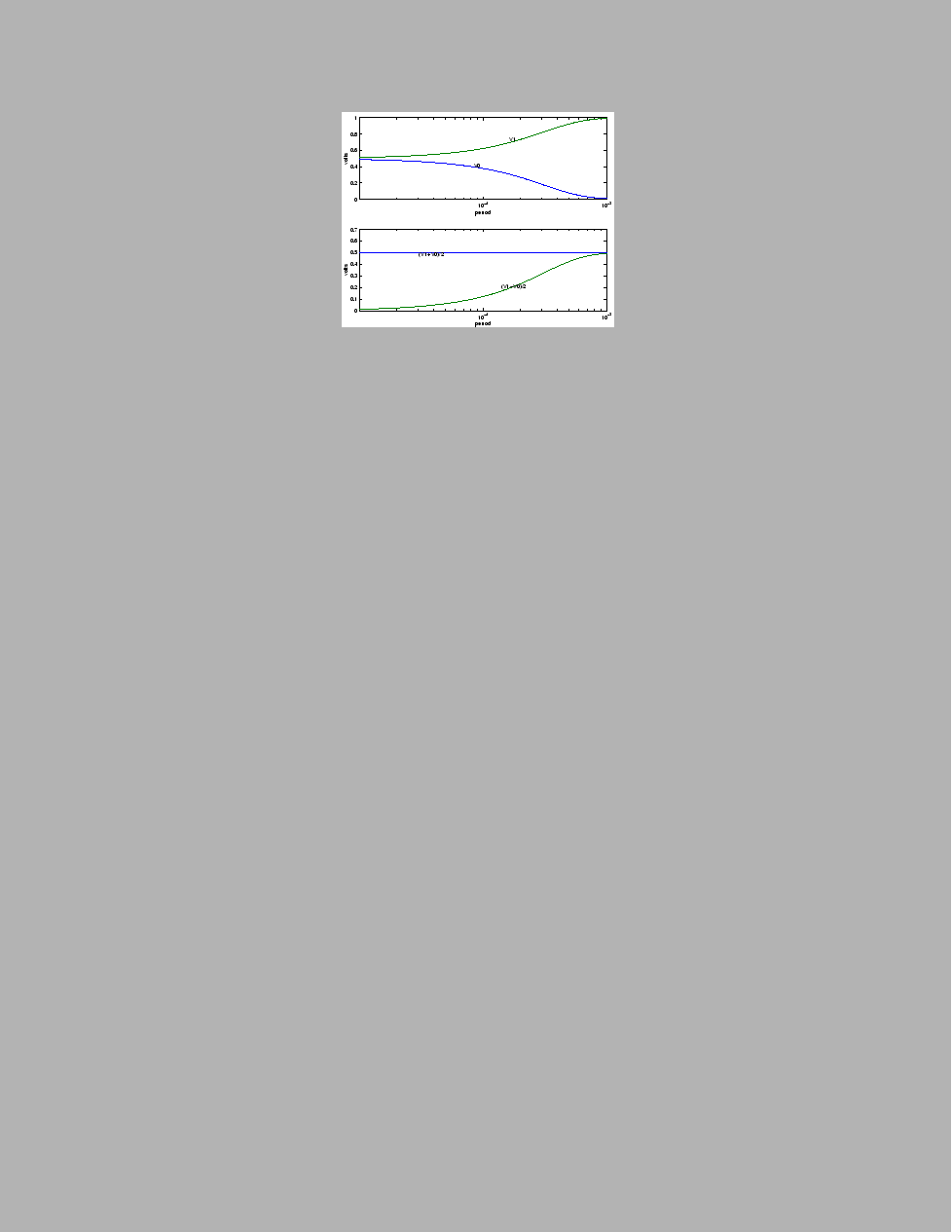, in time, the value of() will always be repeated at regular time intervalsin the future. We refer toas the period of the signal. Ifis the smallest such positive number such that, then we refer toas the signal's fundamental period. Ifis the period of a periodic signal, we often refer toas being-periodic.

A pulse width modulated signal is a-periodic signal,, where there exists a time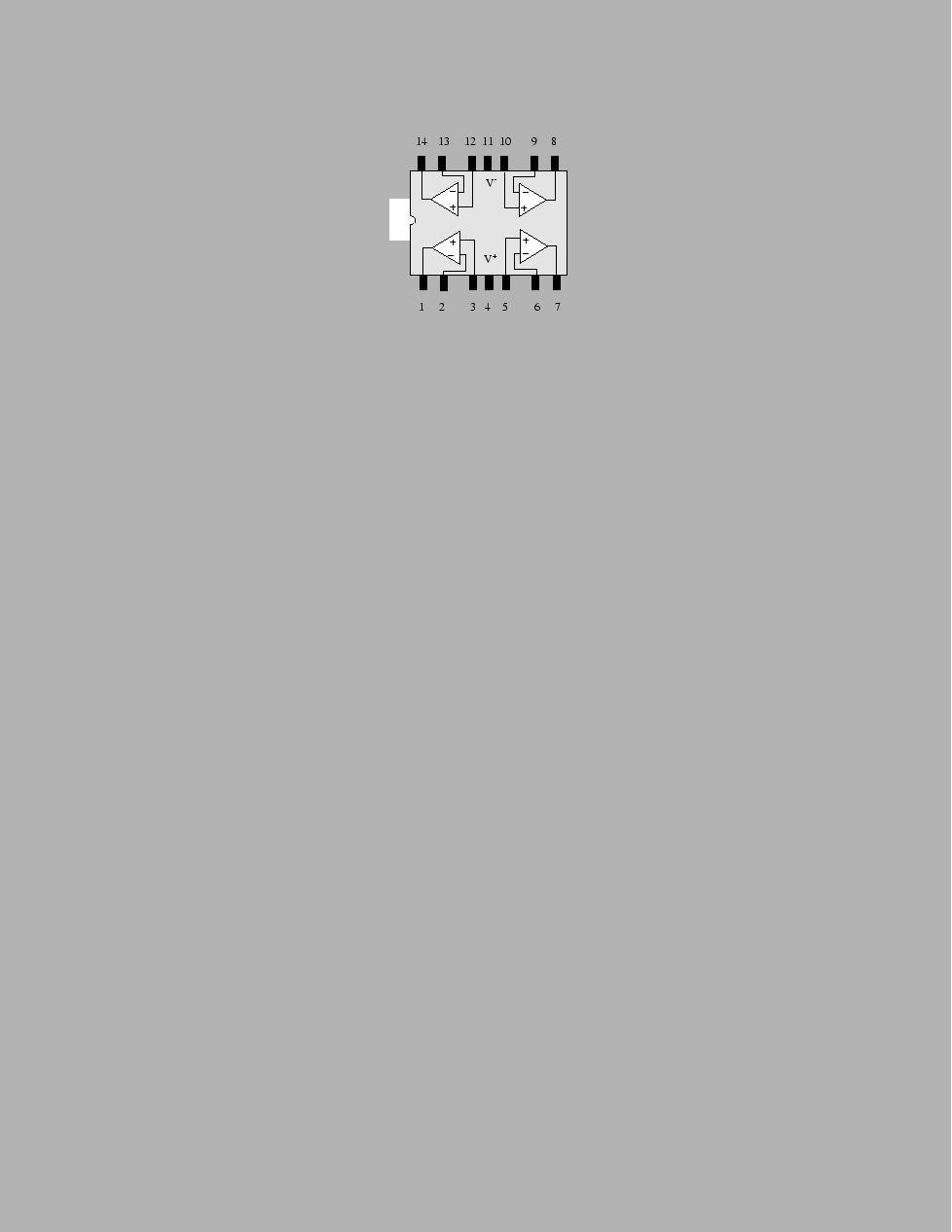such that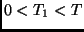and such that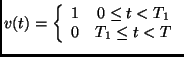(1)

for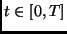. We fer to the ration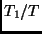as the duty cycle of the signal. We usually represent the duty cycle as a percentage. Equation 1 defines the values thattakes over a single period,. Sinceis-periodic, we know that the pattern characterized in equation 1 will repeat itself at regular intervals of duration. Figure 33 shows a pulse-width modulated signal whose duty cycle is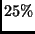.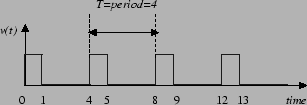Next: How to use a Up: Output Compare Interrupts Previous: Declaring the ISR's Interrupt
Bill Goodwine 2002-09-29